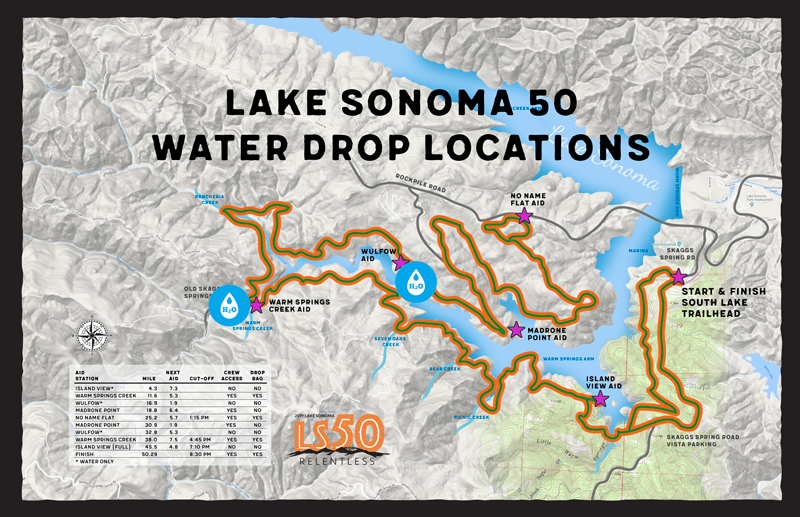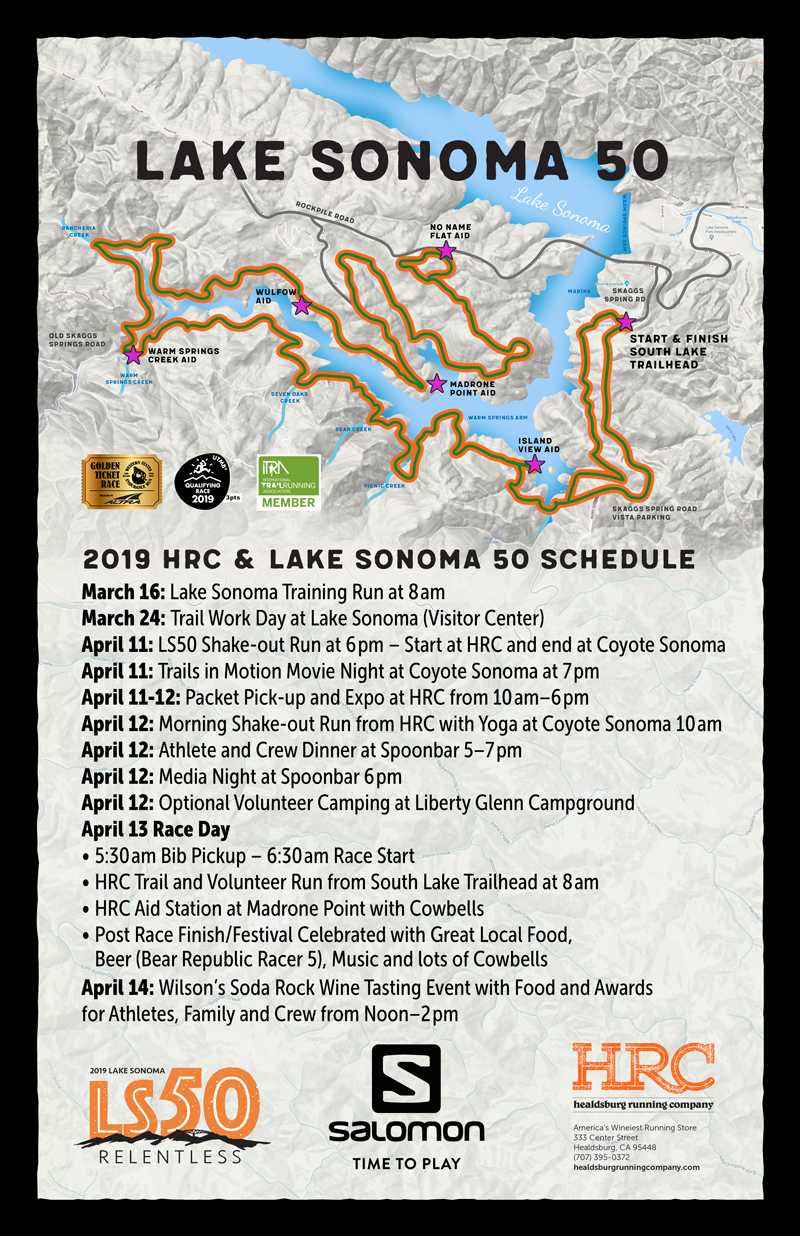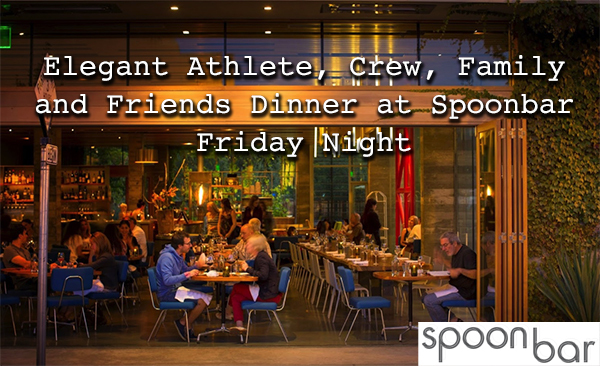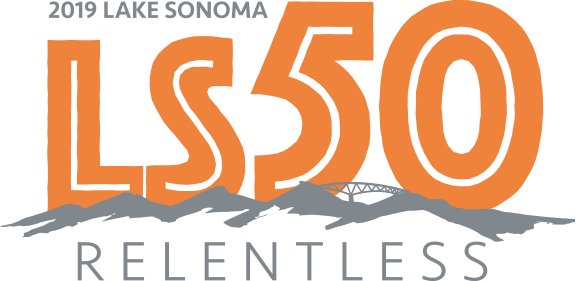/* styles */ Dear Lake Sonoma 50 Athletes, We are less than 3 weeks out from the big day! We hope you are as excited as we are! All that training and preparing is going to come in handy on the course, we can’t wait to share our Tropical year with you. Bring your Tropical shirt!
 table.module-2{width:75.47%;padding:0}table div table+table+table div table{width:75.47%;float:none;margin-left:auto;margin-right:auto;padding:0}table div table+table+table div table a{border:0 none;text-decoration:none}table div table+table+table div table img{width:100%!important;border:0 none;text-decoration:none}table div table+table+table div table td{width:100%;padding:0}/* styles */
 /* styles */ Have you booked your room? This is your last chance to book a room at Hotel Trio with a guaranteed room at the discounted LS50 rate. Having a temporary home set up and secured takes a lot of stress off race week!
 table div table+table+table+table+table div table{width:100%;padding:0}table div table+table+table+table+table div table img{width:96.23%;padding:0;float:none}table div table+table+table+table+table div table td{width:100%;padding:0 1.88% 18px}/* styles *//* styles */ Are you coming into town early, and want to preview the course? We think that’s a great idea, in fact we have water drops along the route to make sure you stay hydrated and safe before the race!
 table div table+table+table+table+table+table+table+table div table{width:100%;padding:0}table div table+table+table+table+table+table+table+table div table img{width:96.23%;padding:0;float:none}table div table+table+table+table+table+table+table+table div table td{width:100%;padding:0 1.88% 18px}/* styles *//* styles */ We have an amazing race week for you this year! Here is a reminder of all the events we have lined up for you to make Lake Sonoma 50 2019 the best year yet! On Thursday, April 11 we have double the fun! At 6 pm we host a shake-out run after packet pick-up that ends with wine & beer at Coyote Sonoma for the Trails in Motion Film Festival. The movie is at 7:30 pm and features a panel with Robert Rhodes and Bob Shebest plus special ultra runner guests! The films are proudly presented by Healdsburg Running Company at no charge, however in lieu of tickets we ask you donate to The Lake Sonoma 50 charity – Children Of Vineyard Workers Scholarship Fund.
 table.module-10{width:27.92%;padding:0}table div table+table+table+table+table+table+table+table+table+table+table div table{width:27.92%;float:none;margin-left:auto;margin-right:auto;padding:0}table div table+table+table+table+table+table+table+table+table+table+table div table a{border:0 none;text-decoration:none}table div table+table+table+table+table+table+table+table+table+table+table div table img{width:100%!important;border:0 none;text-decoration:none}table div table+table+table+table+table+table+table+table+table+table+table div table td{width:100%;padding:0}/* styles */
 table div table+table+table+table+table+table+table+table+table+table+table+table div table td,table.module-11{width:100%;padding:0}table div table+table+table+table+table+table+table+table+table+table+table+table div table{width:100%;float:none;margin-left:auto;margin-right:auto;padding:0}table div table+table+table+table+table+table+table+table+table+table+table+table div table a{border:0 none;text-decoration:none}table div table+table+table+table+table+table+table+table+table+table+table+table div table img{width:100%!important;border:0 none;text-decoration:none}/* styles */
 table div table+table+table+table+table+table+table+table+table+table+table+table+table div table{width:100%;padding:0}table div table+table+table+table+table+table+table+table+table+table+table+table+table div table img{width:96.23%;padding:0;float:none}table div table+table+table+table+table+table+table+table+table+table+table+table+table div table td{width:100%;padding:0 1.88% 18px}/* styles *//* styles */ On Friday, April 12 at 5 pm you are cordially invited to our athlete & crew dinner at Spoonbar in Healdsburg after packet pick-up. Come join us for a night of friends, food, and fun! Spoonbar offers an exceptional menu that is locally sourced and seasonally curated by some of the finest chefs in Wine Country. Relax and refuel before race day and enjoy a panel of guest speakers in the luxurious ambience of Spoonbar.table div table+table+table+table+table+table+table+table+table+table+table+table+table+table+table+table div table{width:100%;padding:0}table div table+table+table+table+table+table+table+table+table+table+table+table+table+table+table+table div table img{width:96.23%;padding:0;float:none}table div table+table+table+table+table+table+table+table+table+table+table+table+table+table+table+table div table td{width:100%;padding:0 1.88% 18px}/* styles *//* styles */ This year we have some stylishly cool hats and Hydro Flask tumblers to commemorate your race! Visit our online store to get yours today! Don’t wait, these limited edition hats and tumblers are quick to sell out!
 table.module-17{width:75.47%;padding:0}table div table+table+table+table+table+table+table+table+table+table+table+table+table+table+table+table+table+table div table{width:75.47%;float:none;margin-left:auto;margin-right:auto;padding:0}table div table+table+table+table+table+table+table+table+table+table+table+table+table+table+table+table+table+table div table a{border:0 none;text-decoration:none}table div table+table+table+table+table+table+table+table+table+table+table+table+table+table+table+table+table+table div table img{width:100%!important;border:0 none;text-decoration:none}table div table+table+table+table+table+table+table+table+table+table+table+table+table+table+table+table+table+table div table td{width:100%;padding:0}/* styles */
 table.module-18{width:75.47%;padding:0}table div table+table+table+table+table+table+table+table+table+table+table+table+table+table+table+table+table+table+table div table{width:75.47%;float:none;margin-left:auto;margin-right:auto;padding:0}table div table+table+table+table+table+table+table+table+table+table+table+table+table+table+table+table+table+table+table div table a{border:0 none;text-decoration:none}table div table+table+table+table+table+table+table+table+table+table+table+table+table+table+table+table+table+table+table div table img{width:100%!important;border:0 none;text-decoration:none}table div table+table+table+table+table+table+table+table+table+table+table+table+table+table+table+table+table+table+table div table td{width:100%;padding:0}/* styles */
 table div table+table+table+table+table+table+table+table+table+table+table+table+table+table+table+table+table+table+table+table div table{width:100%;padding:0}table div table+table+table+table+table+table+table+table+table+table+table+table+table+table+table+table+table+table+table+table div table img{width:96.23%;padding:0;float:none}table div table+table+table+table+table+table+table+table+table+table+table+table+table+table+table+table+table+table+table+table div table td{width:100%;padding:0 1.88% 18px}/* styles *//* styles */ We will have detailed information for you in the next Athlete Newsletter about packet pick-up and race day. For now, run hard, run happy and taper well!